# will rate, thanks! A proton travels with a speed of 5.05 106 m/s at an angle...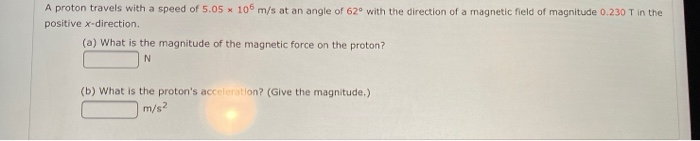will rate, thanks!
A proton travels with a speed of 5.05 106 m/s at an angle of 62° with the direction of a magnetic field of magnitude 0.230 T in the positive x-direction. (a) What is the magnitude of the magnetic force on the proton? N (b) What is the proton's acceleration? (Give the magnitude.) m/s2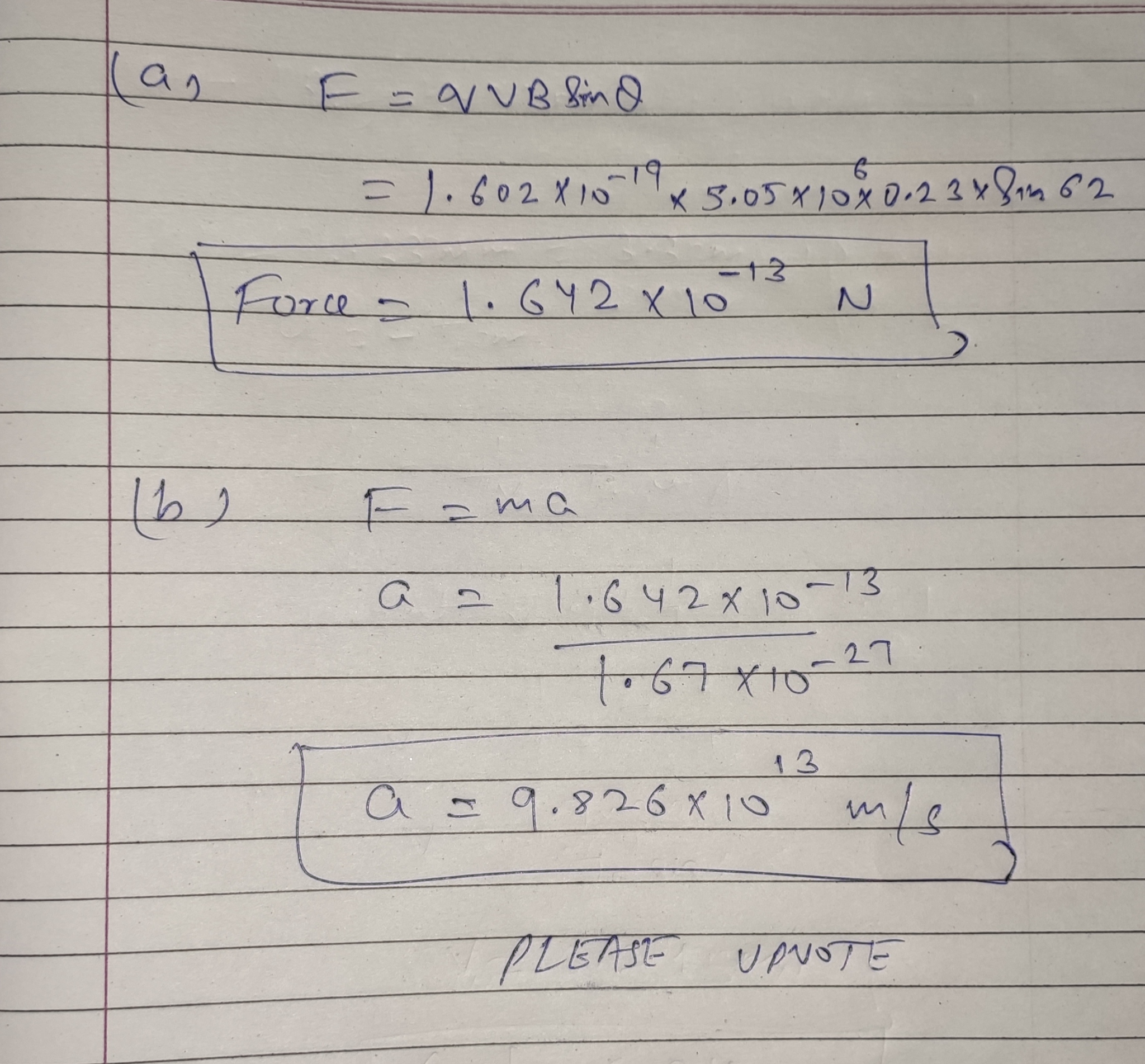#### Earn Coin

Coins can be redeemed for fabulous gifts.

Similar Homework Help Questions
• ### Will rate for answer with work! A proton travels with a speed of 5.00 X 106...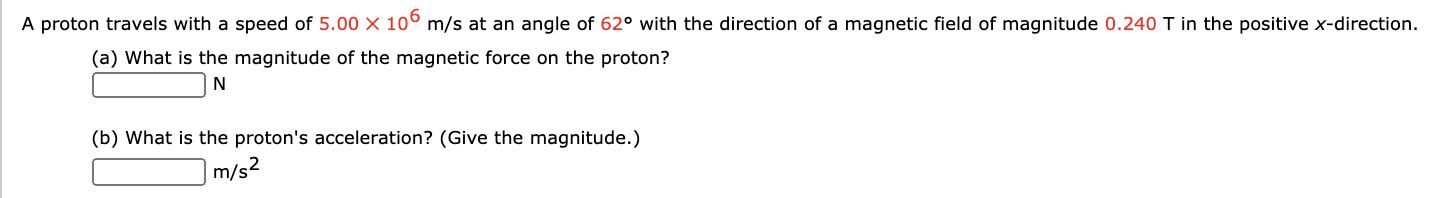Will rate for answer with work! A proton travels with a speed of 5.00 X 106 m/s at an angle of 62° with the direction of a magnetic field of magnitude 0.240 T in the positive x-direction. (a) What is the magnitude of the magnetic force on the proton? N (b) What is the proton's acceleration? (Give the magnitude.) m/s2

• ### A proton travels with a speed of 5.00 x 106 m/s at an angle of 61°...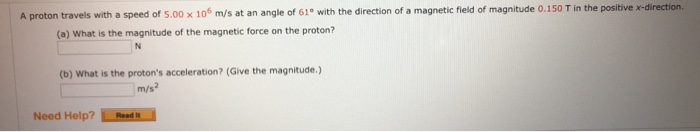A proton travels with a speed of 5.00 x 106 m/s at an angle of 61° with the direction of a magnetic field of magnitude 0.150 T in the positive x-direction. (a) What is the magnitude of the magnetic force on the proton? (b) What is the proton's acceleration? (Give the magnitude.) Need Help? Read

• ### 009 (part 1 of 3) 10.0 points A proton travels with a speed of 4.8×106 m/s...

009 (part 1 of 3) 10.0 points A proton travels with a speed of 4.8×106 m/s at an angle of 96◦ west of north. A magnetic field of 4.6 T points to the north. Find the magnitude of the magnetic force on the proton. (The magnetic force experienced by the proton in the magnetic field is proportional to the component of the proton’s velocity that is perpendicular to the magnetic field.) Answer in units of N. 010 (part 2 of...

• ### PHYSICS HELP!!!! PLZ!

A proton travels with a speed of 4.90 106 m/s at an angle of 64° with the direction of a magnetic field of magnitude 0.250 T in the positive x-direction.(a) What is the magnitude of the magnetic force on the proton?Answer - __________ N(b) What is the proton's acceleration?Answer - __________ m/s2

• ### A proton travels at a uniform speed of 2.50 × 106 m/s toward the right of...

A proton travels at a uniform speed of 2.50 × 106 m/s toward the right of this page. It then enters a uniform magnetic field and experiences a maximum force of 4.95 × 10-8 N that points toward the top of the page. What is the magnitude of the magnetic field?What is the direction of the magnetic field?

• ### 007 (part 1 of 2) 10.0 points A proton travels with a speed of 3.08 x...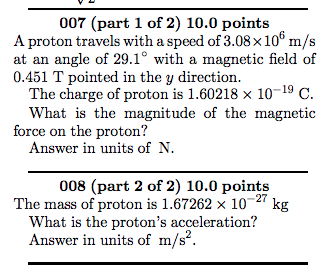007 (part 1 of 2) 10.0 points A proton travels with a speed of 3.08 x 10 m/s at an angle of 29.1° with a magnetic field of 0.451 T pointed in the y direction. The charge of proton is 1.60218 x 10-19 C. What is the magnitude of the magnetic force on the proton? Answer in units of N. 008 (part 2 of 2) 10.0 points The mass of proton is 1.67262 x 10-27 kg What is the proton's...

• ### A proton travels with a speed of 3.00 x 10 m/s at an angle of 34.0°...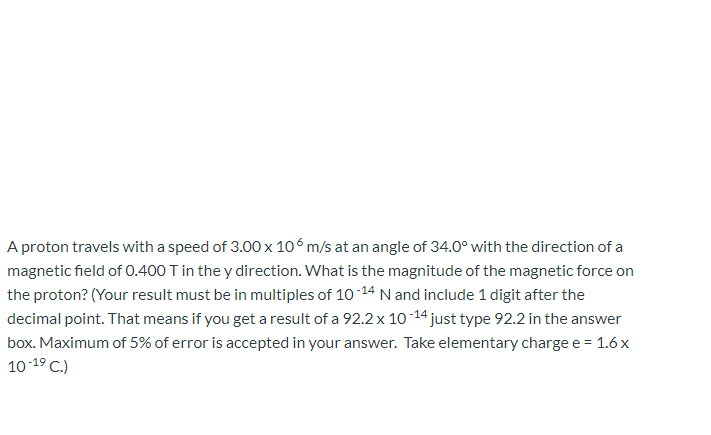A proton travels with a speed of 3.00 x 10 m/s at an angle of 34.0° with the direction of a magnetic field of 0.400 T in the y direction. What is the magnitude of the magnetic force on the proton? (Your result must be in multiples of 10-14 N and include 1 digit after the decimal point. That means if you get a result of a 92.2 x 10-14 just type 92.2 in the answer box. Maximum of 5%...

• ### A proton moving at 6.60 106 m/s through a magnetic field of magnitude 1.78 T experiences...

A proton moving at 6.60 106 m/s through a magnetic field of magnitude 1.78 T experiences a magnetic force of magnitude 7.20 10-13 N. What is the angle between the proton's velocity and the field? (Enter both possible answers from smallest to largest. Enter only positive values between 0 and 360.)

• ### a proton travels with a The City University of New York General Physics 2, Quiz 4...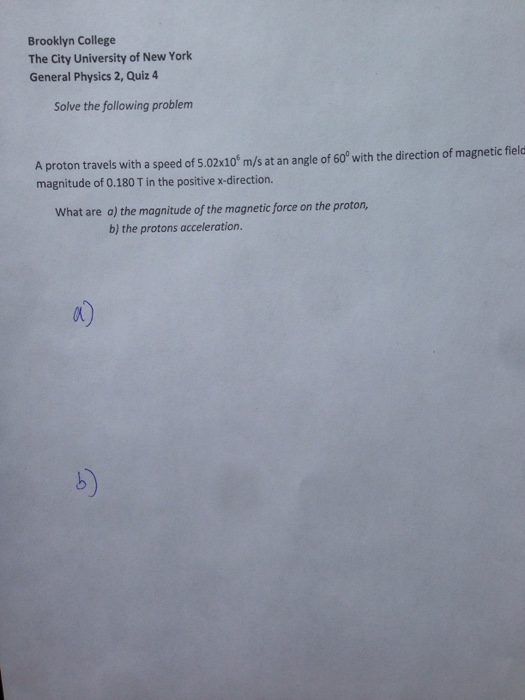a proton travels with a The City University of New York General Physics 2, Quiz 4 A proton travels with a speed of 5.02 times 10^6 m/s at an angle of 60 degree with the direction of magnetic field magnitude of 0.180 T in the positive x-direction. What are a) the magnitude of the magnetic force on the proton, the protons acceleration.

• ### A proton moving at 7.00 x 106 m/s through a magnetic field of magnitude 2.6 T...

A proton moving at 7.00 x 106 m/s through a magnetic field of magnitude 2.6 T experiences magnetic force of magnitude 6.9x10-13 N. What is the angle between the proton's velocity and the field? 6.2° 76.3° 13.7° 44.9°